# Compare Two Lists

This example describes how to compare two lists using conditional formatting. For example, you may have two lists of NFL teams.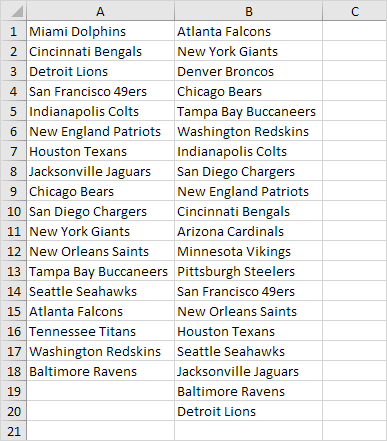To highlight the teams in the first list that are not in the second list, execute the following steps.

1. First, select the range A1:A18 and name it firstList, select the range B1:B20 and name it secondList.

2. Next, select the range A1:A18.

3. On the Home tab, in the Styles group, click Conditional Formatting.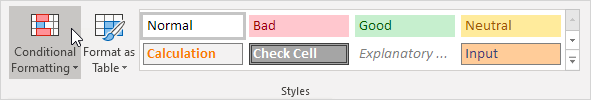4. Click New Rule.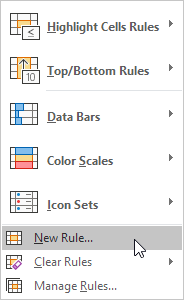5. Select 'Use a formula to determine which cells to format'.

6. Enter the formula =COUNTIF(secondList,A1)=0

7. Select a formatting style and click OK.Result. Miami Dolphins and Tennessee Titans are not in the second list.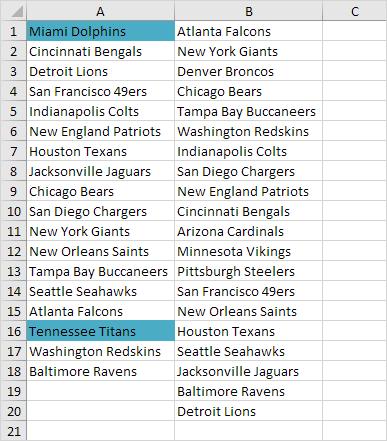Explanation: =COUNTIF(secondList,A1) counts the number of teams in secondList that are equal to the team in cell A1. If COUNTIF(secondList,A1) = 0, the team in cell A1 is not in the second list. As a result, Excel fills the cell with a blue background color. Always write the formula for the upper-left cell in the selected range (A1:A18). Excel automatically copies the formula to the other cells. Thus, cell A2 contains the formula =COUNTIF(secondList,A2)=0, cell A3 =COUNTIF(secondList,A3)=0, etc.

8. To highlight the teams in the second list that are not in the first list, select the range B1:B20, create a new rule using the formula =COUNTIF(firstList,B1)=0, and set the format to orange fill.

Result. Denver Broncos, Arizona Cardinals, Minnesota Vikings and Pittsburgh Steelers are not in the first list.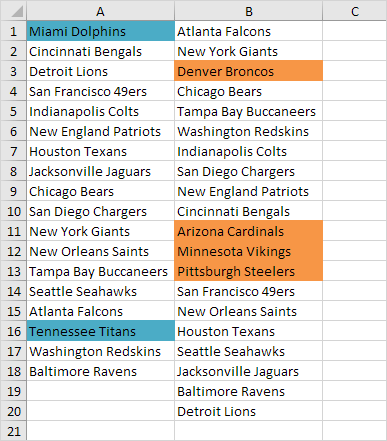Go to Next Chapter: Charts